# Further Statistics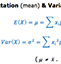Statistics 2 Formula (Part 2) (PDF here) This set of notes covers lots of the further maths Stats topics.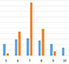Central Limit Theorem Graphic demonstration of the Central Limit Theorem. Use sliders to change shapes of graphs. Two tabs - one to demonstrate non-normal distributions, one to demonstrate standard deviation of estimations of normal distributions.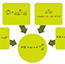Poisson Diagram Visual diagram highlighting key maths contributory ideas to the Poisson Distribution.Statistics Distributions Summary document highlighting key features, criteria, examples, notation and equations of the binomial, normal, poisson and geometric distributions. PDF here.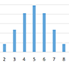Graphs of Distributions Dynamic graphs of the binomial, normal and poisson distributions. Use sliders to change values and shapes.Identifying the Reference Distribution Notes on features to consider when deciding which distribution to use for hypothesis testing. Pdf version. Tree Diagram.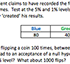Chi-Squared Concept Questions A set of questions designed to assess students understanding of the chi-squared hypothesis test. These questions all involve 'single' sets of data, in preparation for contingency tables later on.Trying to be Random Imagine rolling the die 40 times, what may the results be? Then carry out a chi-squared test to assess your randomness.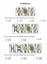Combinations How many ways to choose 0, 1, 2 or 3 from 3 cards. Or 0-4 from 4 cards, or even 5! (An introduction to Pascals).Perms & Combs Crib sheet for Stats 1 - Permutations and Combinations (PDF here)